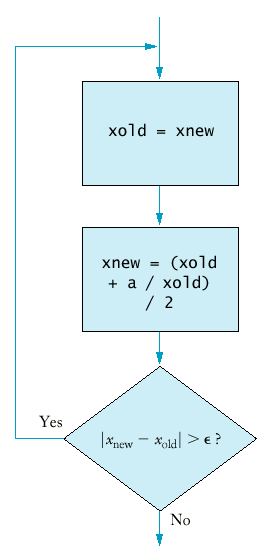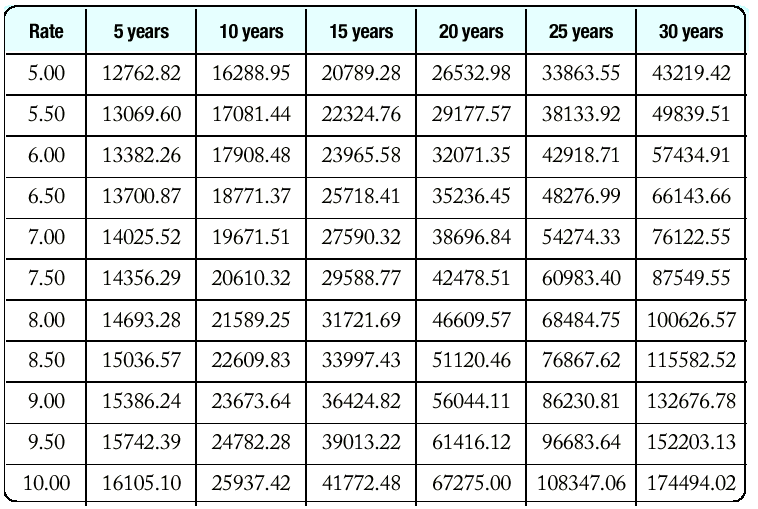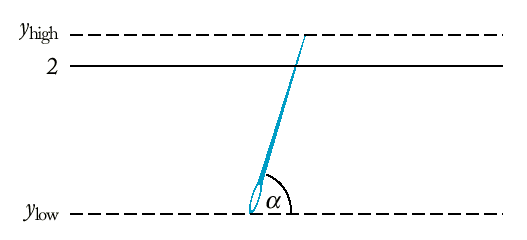## Chapter Goals

• To be able to program loops with the do/while statement
• To learn how to process character, word, and line input
• To learn how to read input from a file through redirection
• To implement approximations and simulations

## The do Loop

• Sometimes you want to execute the body of a loop at least once and perform the loop test after the body was executed.
• The do/while loop serves that purpose.
`do{ statements}while (condition);`
• Example: A simple approximation algorithm to compute square root (ancient Greeks) - the sequence

x0 = axn+1 = ( xn + a/xn ) / 2
converges and has limit square root of a.
`do{  xold = xnew;   xnew = (xold + a / xold) / 2;}while (fabs(xnew - xold) > EPSILON);`
Stop when two successive approximations differ from each other by a very small amount. We want to enter the loop at least once so that we can compute the difference between two approximations.## The do Loop (Syntax 7.2: do/while Statement)

Syntax 7.2: do/while Statement

`do statement while (condition);`
 Example: `do x = sqrt(x); while (x >= 10);` Purpose: Execute the statement, then test the condition, and repeat the statement while the condition remains true.

## Nested Loops

• How can we print a table of values?
• For example, this table tells you the fate of \$10,000 invested under various interest rates for a different number of years.• Here is the pseudocode:
`print table headerdouble rate;for (rate = RATE_MIN; rate <= RATE_MAX;      rate = rate + RATE_INCR){  print table row}`
• How do we print a table row? You need to program another loop.
`int year;for (year = YEAR_MIN; year <= YEAR_MAX;     year = year + YEAR_INCR){  balance = future_value(initial_balance, rate, year);   cout << setw(10) << balance;}`
• It's a good idea to print the table header as a loop as well.
`cout << "Rate     ";int year;for (year = YEAR_MIN; year <= YEAR_MAX;     year = year + YEAR_INCR){  cout << setw(2) << year << " years";}`
• Once everything is put together, we have the loop printing a single row nested in the loop that traverses the interest rates.

## Nested Loops

• Sometimes the iteration count of the inner loop depends on the outer loop.
• Example: printing a triangle shape.
`[][][][][][][][][][]`
• To print n rows, use the loop.
`for (int i = 1; i <= n; i++){  print triangle row}`
• Each row contains i boxes.
`for (int j = 1; j <= i; j++) cout << "[]";cout << "\n";`
• Putting the two loops together yields
`for (int i = 1; i <= n; i++){  for (int j = 1; j <= i; j++) cout << "[]";   cout << "\n";}`
• triangle.cpp

## Processing Text Input

• When processing text input we need to make a decision - is the input structured as a sequence of characters, words, or lines?
• To input a sequence of word, use the loop
`string word;while (cin >> word){  process word}`
• cin >> word has the same value as cin - it is the same test as !cin.fail().
• To process input one line at a time, use the getline function.
`string line;while (getline(cin, line)){  process line}`
• As above, the getline function returns cin, so you can test that cin hasn't yet failed.
• To process input character by character, use the loop
`char ch;while (cin.get(ch)){  process ch}`

## Processing Text Input (words.cpp)

• You would type text in, and at the end of the input the program would tell you how many words you typed. (You would never want to use such a program!)
• The program makes a lot of sense if input is read from a file.
• To link a file to the input of a program you type
`words < article.txt`
on a command line. The input instructions no longer expect input from the keyboard but from the file article.txt.
• Input redirection on the command line.
• You can redirect also output of this program typing
`words < article.txt > output.txt`

## Simulations

• In a simulation we generate random events and evaluate their outcomes.
• The C++ library has a random number generator, which produces numbers that appear to be random.
• Calling rand() yields a random number between 0 and RAND_MAX (usually 32767, but implementation dependent).
• These numbers actual come from a a very long sequence of numbers computed from fairly simple formulas; they just behave like random numbers. For that reason they are often called pseudorandom numbers.
• The following program produces the exact same output every time the program is run (because the numbers are generated with formulas).
`int main(){  int i;   for (i = 1; i <= 10; i++)   {  int r = rand();      cout << r << "\n";   }   return 0;}`
• When you specify a new seed for the random number sequence, the generator generates a new (different) sequence.The seed is set with the srand function.
• By setting the seed based on the time, the random numbers will be different every time you run the program.
`Time now;int seed = now.seconds_from(Time(0,0,0));srand(seed);`
• In actual applications you want to generate random numbers in different ranges, say between a and b.
• For example, to simulate a die roll, you need numbers between a = 1 and b = 6.
• First compute rand() % (b - a + 1) to obtain a random value between 0 and b - a.
• Add that to a to yield a random value between a and b.
`int rand_int(int a, int b){  return a + rand() % (b - a + 1);}`

## Simulations (dice.cpp)

• To generate a random floating-point number, note that the quantity rand() * 1.0 / RAND_MAX is a random floating-point value between 0 and 1.
• The 1.0 ensures that the division is a floating-point number.
`double rand_double(double a, double b){  return a + (b - a) * rand() * (1.0 / RAND_MAX);}`

## Simulations (Buffon Needle Experiment)

• Devised by Comte George-Louis Leclerc de Buffon (1707-1788), a French naturalist.
• A needle of length 1 inch is dropped onto paper that is ruled with parallel lines 2 inches apart.
• If the needle drops onto a line, we count it as a hit.
• The quotient tries / hits approximates pi.
• Our program randomly generates the lower point of the needle and an angle.
• The upper point of the needle is given by yhigh = ylow + sin(angle).
• If yhigh >= 2, we count the needle as a hit.## Simulations (buffon.cpp)

• The point of this program is not to compute pi (after all, we needed the value of pi in the deg2rad function). Rather, the point is to show how to a physical experiment can be simulated on the computer.